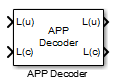Documentation

# APP Decoder

Decode convolutional code using a posteriori probability (APP) method

## Library

Convolutional sublibrary of Error Detection and Correction## Description

The APP Decoder block performs a posteriori probability (APP) decoding of a convolutional code.

### Input Signals and Output Signals

The input L(u) represents the sequence of log-likelihoods of encoder input bits, while the input L(c) represents the sequence of log-likelihoods of code bits. The outputs L(u) and L(c) are updated versions of these sequences, based on information about the encoder.

If the convolutional code uses an alphabet of 2n possible symbols, this block's L(c) vectors have length Q*n for some positive integer Q. Similarly, if the decoded data uses an alphabet of 2k possible output symbols, then this block's L(u) vectors have length Q*k.

This block accepts a column vector input signal with any positive integer for Q.

If you only need the input L(c) and output L(u), you can attach a Simulink Ground block to the input L(u) and a Simulink® Terminator block to the output L(c).

This block accepts `single` and `double` data types. Both inputs, however, must be of the same type. The output data type is the same as the input data type.

### Specifying the Encoder

To define the convolutional encoder that produced the coded input, use the Trellis structure parameter. This parameter is a MATLAB® structure whose format is described in Trellis Description of a Convolutional Code. You can use this parameter field in two ways:

• If you have a variable in the MATLAB workspace that contains the trellis structure, enter its name as the Trellis structure parameter. This way is preferable because it causes Simulink to spend less time updating the diagram at the beginning of each simulation, compared to the usage described next.

• If you want to specify the encoder using its constraint length, generator polynomials, and possibly feedback connection polynomials, use a `poly2trellis` command within the Trellis structure field. For example, to use an encoder with a constraint length of 7, code generator polynomials of 171 and 133 (in octal numbers), and a feedback connection of 171 (in octal), set the Trellis structure parameter to

`poly2trellis(7,[171 133],171)`

To indicate how the encoder treats the trellis at the beginning and end of each frame, set the Termination method parameter to either `Truncated` or `Terminated`. The `Truncated` option indicates that the encoder resets to the all-zeros state at the beginning of each frame. The `Terminated` option indicates that the encoder forces the trellis to end each frame in the all-zeros state. If you use the Convolutional Encoder block with the Operation mode parameter set to ```Truncated (reset every frame)```, use the `Truncated` option in this block. If you use the Convolutional Encoder block with the Operation mode parameter set to ```Terminate trellis by appending bits```, use the `Terminated` option in this block.

### Specifying Details of the Algorithm

You can control part of the decoding algorithm using the Algorithm parameter. The ```True APP``` option implements a posteriori probability decoding as per equations 20–23 in section V of . To gain speed, both the `Max*` and `Max` options approximate expressions like

`$\mathrm{log}\sum _{i}\mathrm{exp}\left({a}_{i}\right)$`

by other quantities. The `Max` option uses max(ai) as the approximation, while the `Max*` option uses max(ai) plus a correction term given by $\mathrm{ln}\left(1+\mathrm{exp}\left(-|{a}_{i-1}-{a}_{i}|\right)\right)$ .

The `Max*` option enables the Scaling bits parameter in the dialog box. This parameter is the number of bits by which the block scales the data it processes internally (multiplies the input by (2^`numScalingBits`) and divides the pre-output by the same factor). Use this parameter to avoid losing precision during the computations.

## Parameters

Trellis structure

MATLAB structure that contains the trellis description of the convolutional encoder.

Termination method

Either `Truncated` or `Terminated`. This parameter indicates how the convolutional encoder treats the trellis at the beginning and end of frames.

Algorithm

Either `True APP`, `Max*`, or `Max`.

Number of scaling bits

An integer between 0 and 8 that indicates by how many bits the decoder scales data in order to avoid losing precision. This field is active only when Algorithm is set to `Max*`.

Disable L(c) output port

Select this check box to disable the secondary block output, L(c).

## Examples

For an example using this block, see the Iterative Decoding of a Serially Concatenated Convolutional Code example.

## References

 Benedetto, S., G. Montorsi, D. Divsalar, and F. Pollara, “A Soft-Input Soft-Output Maximum A Posterior (MAP) Module to Decode Parallel and Serial Concatenated Codes,” JPL TDA Progress Report, Vol. 42-127, November 1996.

 Benedetto, Sergio and Guido Montorsi, “Performance of Continuous and Blockwise Decoded Turbo Codes.” IEEE Communications Letters, Vol. 1, May 1997, 77–79.

 Viterbi, Andrew J., “An Intuitive Justification and a Simplified Implementation of the MAP Decoder for Convolutional Codes,” IEEE Journal on Selected Areas in Communications, Vol. 16, February 1998, 260–264.